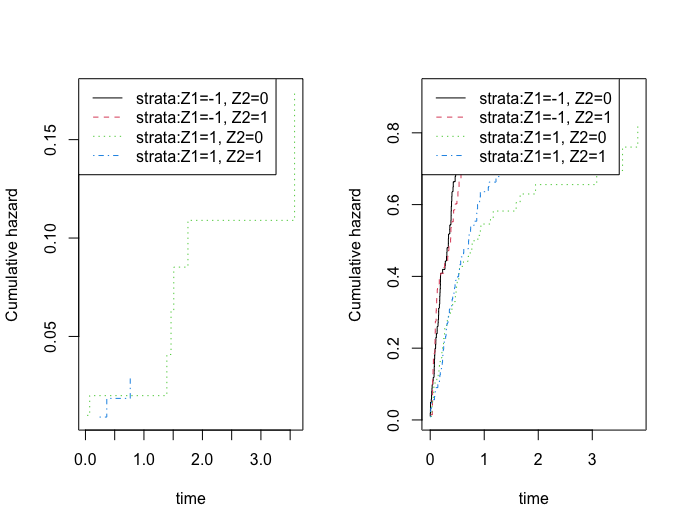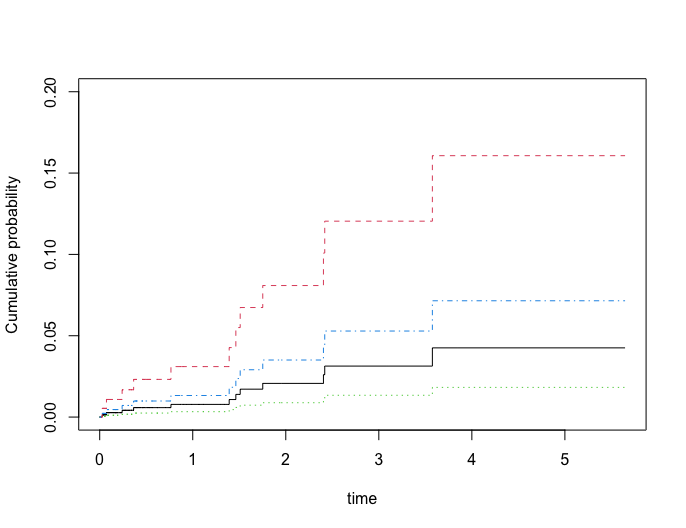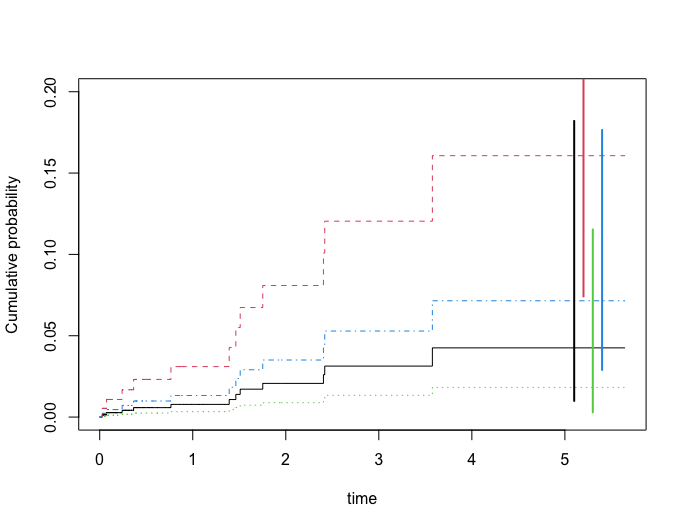# Fine-Gray model

considered \begin{align*} U^{FG}_{n}(\beta) = \sum_{i=0}^{n} \int_0^{+\infty} \left( X_i- E_n(t,\beta) \right) w_i(t,X_i) dN_{1,i}(t) \text{ where } E_n(t,\beta)=\frac{\tilde S_1(t,\beta) }{\tilde S_0(t,\beta)}, \end{align*} with $$w_i(t,X_i) = \frac{G_c(t,X_i)}{G_c(T_i \wedge t,X_i)} I( C_i > T_i \wedge t )$$ ,$$\tilde S_k(t,\beta) = \sum_{j=1}^n X_j^k \exp(X_j^T\beta) Y_{1,j}(t)$$ for $$k=0,1$$, and with with $$\tilde Y_{1,i}(t) = Y_{1,i}(t) w_i(t,X_i)$$ for $$i=1,...,n$$. $$w_i(t)$$ needs to be replaced by an estimator of the censoring distribution, and since it does not depend on $$X$$ the $$\hat w_i(t) = \frac{\hat G_c(t,X_i)}{\hat G_c(T_i \wedge t,X_i)} I(C_i > T_i \wedge t)$$ where $$\hat G_c$$ is the Kaplan-Meier estimator of the censoring distribution.

In this article we briefly introduce some functions for doing cumulative incidence regression, and how to augment the Fine-Gray estimator.

First we simulate some competing risks data using some utility functions.

We simulate data with two causes based on the Fine-Gray model: \begin{align} F_1(t,X) & = P(T\leq t, \epsilon=1|X)=( 1 - exp(-\Lambda_1(t) \exp(X^T \beta_1))) \\ F_2(t,X) & = P(T\leq t, \epsilon=2|X)= ( 1 - exp(-\Lambda_2(t) \exp(X^T \beta_2))) \cdot (1 - F_1(\infty,X)) \end{align} where the baselines are given as $$\Lambda_j(t) = \rho_j (1- exp(-t/\nu_j))$$ for $$j=1,2$$, and the $$X$$ being two independent binomials. Alternatively, one can also replace the FG-model with a logistic link $$\mbox{expit}( \Lambda_j(t) + \exp(X^T \beta_j))$$.

The advantage of the model is that it is easy to fit and to get standard errors, and that it is quite flexible essentially being a Cox-model. On the downside is that the coefficients are quite hard to interpret since they are the $$cloglog$$ coefficients of $$1-F_1(t,X)$$. Specifically, \begin{align} \log(-\log( 1-F_1(t,X_1+1,X_2))) - \log(-\log( 1-F_1(t,X_1,X_2))) & = \beta_1, \end{align} so the effect is $$\beta_1$$ of $$X_1$$ is on $$1-F_1(t,X)$$ on the $$cloglog$$ scale.

 library(mets)
options(warn=-1)
set.seed(1000) # to control output in simulatins for p-values below.

rho1 <- 0.2; rho2 <- 10
n <- 400
beta=c(0.0,-0.1,-0.5,0.3)
## beta1=c(0.0,-0.1); beta2=c(-0.5,0.3)
dats <- simul.cifs(n,rho1,rho2,beta,rc=0.5,rate=7)
dtable(dats,~status)
#>
#> status
#>   0   1   2
#> 127  12 261
dsort(dats) <- ~time

We have a look at the non-parametric cumulative incidence curves

 par(mfrow=c(1,2))
cifs1 <- cif(Event(time,status)~strata(Z1,Z2),dats,cause=1)
plot(cifs1)

cifs2 <- cif(Event(time,status)~strata(Z1,Z2),dats,cause=2)
plot(cifs2)Now fitting the Fine-Gray model

 fg <- cifreg(Event(time,status)~Z1+Z2,data=dats,cause=1,propodds=NULL)
summary(fg)
#>
#>    n events
#>  400     12
#>
#>  400 clusters
#> coeffients:
#>    Estimate     S.E.  dU^-1/2 P-value
#> Z1  0.69686  0.38760  0.38882  0.0722
#> Z2 -0.85929  0.62453  0.61478  0.1689
#>
#> exp(coeffients):
#>    Estimate    2.5%  97.5%
#> Z1  2.00744 0.93911 4.2911
#> Z2  0.42346 0.12451 1.4402

dd <- expand.grid(Z1=c(-1,1),Z2=0:1)
pfg <- predict(fg,dd)
plot(pfg,ylim=c(0,0.2))# SE’s for the baseline and predictions of FG

The standard errors reported for the FG-estimator are based on the i.i.d decompostion (influence functions) of the estimator that we give later. A similar decompostion exist for the baseline and is needed when standard errors of predictions are computed. These are a bit harder to compute for all time-points simultaneously, but they can be obtained for specific timepoints jointly with the iid decomposition of the regression coefficients and then used to get standard errors for predictions.

We here plot the predictions with jittered confidence intervals for the predictions at time point 5

### predictions with CI based on iid decomposition of baseline and beta
fg <- cifreg(Event(time,status)~Z1+Z2,data=dats,cause=1,propodds=NULL,cox.prep=TRUE)
Biid <- IIDbaseline.cifreg(fg,time=5)
pfgse <- FGprediid(Biid,dd)
pfgse
#>            pred    se-log       lower     upper
#> [1,] 0.04253879 0.7418354 0.009938793 0.1820692
#> [2,] 0.16069100 0.3946377 0.074143886 0.3482633
#> [3,] 0.01823957 0.9410399 0.002884032 0.1153531
#> [4,] 0.07149610 0.4611261 0.028958169 0.1765199
plot(pfg,ylim=c(0,0.2))
for (i in 1:4) lines(c(5,5)+i/10,pfgse[i,3:4],col=i,lwd=2)The iid decompostions are stored inside Biid, in addition we note that the iid decompostions for $$\hat \beta - \beta_0$$ are obtained by the command iid()

# Comparison

We compare with the cmprsk function but without running it to avoid dependencies:

run <- 0
if (run==1) {
library(cmprsk)
mm <- model.matrix(~Z1+Z2,dats)[,-1]
cr <- with(dats,crr(time,status,mm))
cbind(cr$coef,diag(cr$var)^.5,fg$coef,fg$se.coef,cr$coef-fg$coef,diag(cr$var)^.5-fg$se.coef)
#          [,1]      [,2]       [,3]      [,4]          [,5]          [,6]
# Z1  0.6968603 0.3876029  0.6968603 0.3876029 -1.534155e-09 -7.811395e-10
# Z2 -0.8592892 0.6245258 -0.8592892 0.6245258  8.537615e-14  4.430734e-11
}

if (run==1) {
fg0 <- cifreg(Event(time,status)~Z1+Z2,data=dats,cause=1,propodds=NULL,beta=cr$coef,no.opt=TRUE) cbind(diag(cr$var)^.5,fg0$se.coef,diag(cr$var)^.5-fg0$se.coef) # [,1] [,2] [,3] # [1,] 0.3876029 0.3876029 -8.881784e-16 # [2,] 0.6245258 0.6245258 3.330669e-16 } When comparing with the results from the coxph based on setting up the data using the finegray function, we get the same estimates but note that the standard errors of the coxph is missing a term and therefore slightly different. When comparing to the estimates from coxph missing the additional censoring term we see that we get also the same standard errors if (run==1) { dats$id <- 1:nrow(dats)
dats$event <- factor(dats$status,0:2, labels=c("censor", "death", "other"))
fgdats <- finegray(Surv(time,event)~.,data=dats)
coxfg <- coxph(Surv(fgstart, fgstop, fgstatus) ~ Z1+Z2 + cluster(id), weight=fgwt, data=fgdats)

fg0 <- cifreg(Event(time,status)~Z1+Z2,data=dats,cause=1,propodds=NULL)
cbind( coxfg$coef,fg0$coef, coxfg$coef-fg0$coef)
#          [,1]       [,2]          [,3]
# Z1  0.6968603  0.6968603 -1.534153e-09
# Z2 -0.8592892 -0.8592892  8.726353e-14
cbind(diag(coxfg$var)^.5,fg0$se.coef,diag(coxfg$var)^.5-fg0$se.coef)
#           [,1]      [,2]          [,3]
# [1,] 0.3889129 0.3876029  0.0013099907
# [2,] 0.6241225 0.6245258 -0.0004033148
cbind(diag(coxfg$var)^.5,fg0$se1.coef,diag(coxfg$var)^.5-fg0$se1.coef)
#           [,1]      [,2]          [,3]
# [1,] 0.3889129 0.3889129 -7.839487e-10
# [2,] 0.6241225 0.6241225  4.448153e-11
}

We also remove all censorings from the data to compare the estimates with those based on coxph, and observe that the estimates as well as the standard errors agree

datsnc <- dtransform(dats,status=2,status==0)
dtable(datsnc,~status)
#>
#> status
#>   1   2
#>  12 388
datsnc$id <- 1:n datsnc$entry <- 0
max <- max(dats$time)+1 ## for cause 2 add risk interaval datsnc2 <- subset(datsnc,status==2) datsnc2 <- transform(datsnc2,entry=time) datsnc2 <- transform(datsnc2,time=max) datsncf <- rbind(datsnc,datsnc2) # cifnc <- cifreg(Event(time,status)~Z1+Z2,data=datsnc,cause=1,propodds=NULL) cc <- coxph(Surv(entry,time,status==1)~Z1+Z2+cluster(id),datsncf,robust=TRUE) cbind(cifnc$coef,cifnc$se.coef, cc$coef, diag(cc$var)^.5) #> [,1] [,2] [,3] [,4] #> Z1 0.7376223 0.3962877 0.7376223 0.3962877 #> Z2 -0.8211457 0.6308807 -0.8211457 0.6308807 cbind(cifnc$coef-cc$coef, diag(cc$var)^.5-cifnc$se.coef) #> [,1] [,2] #> Z1 2.053288e-09 -1.048035e-09 #> Z2 1.940670e-13 7.571888e-11 the cmprsk gives something slightly-slightly different if (run==1) { library(cmprsk) mm <- model.matrix(~Z1+Z2,datsnc)[,-1] cr <- with(datsnc,crr(time,status,mm)) cbind(cifnc$coef-cr$coef, diag(cr$var)^.5-cifncse.coef) # [,1] [,2] # Z1 2.053291e-09 -1.048037e-09 # Z2 1.926237e-13 7.571876e-11 } # Strata dependent Censoring weights We can improve efficiency and avoid bias by allowing the censoring weights to depend on the covariates  fgcm <- cifreg(Event(time,status)~Z1+Z2,data=dats,cause=1,propodds=NULL, cens.model=~strata(Z1,Z2)) summary(fgcm) #> #> n events #> 400 12 #> #> 400 clusters #> coeffients: #> Estimate S.E. dU^-1/2 P-value #> Z1 0.54277 0.37188 0.39352 0.1444 #> Z2 -0.91846 0.61886 0.61447 0.1378 #> #> exp(coeffients): #> Estimate 2.5% 97.5% #> Z1 1.72077 0.83019 3.5667 #> Z2 0.39913 0.11867 1.3424 summary(fg) #> #> n events #> 400 12 #> #> 400 clusters #> coeffients: #> Estimate S.E. dU^-1/2 P-value #> Z1 0.69686 0.38760 0.38882 0.0722 #> Z2 -0.85929 0.62453 0.61478 0.1689 #> #> exp(coeffients): #> Estimate 2.5% 97.5% #> Z1 2.00744 0.93911 4.2911 #> Z2 0.42346 0.12451 1.4402 We note that the standard errors are slightly smaller for the more efficient estimator. The influence functions of the FG-estimator is given by , \begin{align*} \phi_i^{FG} & = \int (X_i- e(t)) \tilde w_i(t) dM_{i1}(t,X_i) + \int \frac{q(t)}{\pi(t)} dM_{ic}(t), \\ & = \phi_i^{FG,1} + \phi_i^{FG,2}, \end{align*} where the first term is what would be achieved for a known censoring distribution, and the second term is due to the variability from the Kaplan-Meier estimator. Where $$M_{ic}(t) = N_{ic}(t) - \int_0^t Y_i(s) d\Lambda_c (s)$$ with $$M_{ic}$$ the standard censoring martingale. The function $$q(t)$$ that reflects that the censoring only affects the terms related to cause “2” jumps, can be written as (see Appendix B2) \begin{align*} q(t) & = E( H(t,X) I(T \leq t, \epsilon=2) I(C > T)/G_c(T)) = E( H(t,X) F_2(t,X) ), \end{align*} with $$H(t,X) = \int_t^{\infty} (X- e(s)) G(s) d \Lambda_1(s,X)$$ and since $$\pi(t)=E(Y(t))=S(t) G_c(t)$$. In the case where the censoring weights are stratified (based on $$X$$) we get the influence functions related to the censoring term with \begin{align*} q(t,X) & = E( H(t,X) I(T \leq t, \epsilon=2) I(T < C)/G_c(T,X) | X) = H(t,X) F_2(t,X), \end{align*} so that the influence function becomes \begin{align*} \int (X-e(t)) w(t) dM_1(t,X) + \int H(t,X) \frac{F_2(t,X)}{S(t,X)} \frac{1}{G_c(t,X)} dM_c(t,X). \end{align*} with $$H(t,X) = \int_t^{\infty} (X- e(s)) G(s,X) d \Lambda_1(s,X)$$. # Augmenting the FG-estimator Rather than using a larger censoring model we can also compute the augmentation term directly and then fit the FG-model based on this augmentation term and do a couple of iterations  fgaugS <- FG_AugmentCifstrata(Event(time,status)~Z1+Z2+strata(Z1,Z2),data=dats,cause=1,E=fgE)
summary(fgaugS)
#>
#>    n events
#>  400     12
#>
#>  400 clusters
#> coeffients:
#>    Estimate     S.E.  dU^-1/2 P-value
#> Z1  0.55614  0.29861  0.35607  0.0625
#> Z2 -0.88438  0.61209  0.62011  0.1485
#>
#> exp(coeffients):
#>    Estimate    2.5%  97.5%
#> Z1  1.74392 0.97128 3.1312
#> Z2  0.41297 0.12442 1.3707

fgaugS2 <- FG_AugmentCifstrata(Event(time,status)~Z1+Z2+strata(Z1,Z2),data=dats,cause=1,E=fgaugS$E) summary(fgaugS2) #> #> n events #> 400 12 #> #> 400 clusters #> coeffients: #> Estimate S.E. dU^-1/2 P-value #> Z1 0.56000 0.29957 0.35687 0.0616 #> Z2 -0.88347 0.61172 0.61993 0.1487 #> #> exp(coeffients): #> Estimate 2.5% 97.5% #> Z1 1.75068 0.97321 3.1492 #> Z2 0.41335 0.12463 1.3709 fgaugS3 <- FG_AugmentCifstrata(Event(time,status)~Z1+Z2+strata(Z1,Z2),data=dats,cause=1,E=fgaugS2$E)
summary(fgaugS3)
#>
#>    n events
#>  400     12
#>
#>  400 clusters
#> coeffients:
#>    Estimate     S.E.  dU^-1/2 P-value
#> Z1  0.55989  0.29954  0.35685  0.0616
#> Z2 -0.88350  0.61173  0.61994  0.1487
#>
#> exp(coeffients):
#>    Estimate    2.5%  97.5%
#> Z1  1.75047 0.97317 3.1487
#> Z2  0.41333 0.12462 1.3709

Again we note slightly smaller standard errors when augmenting the estimator.

The function compute the augmentation term for fixed $$E(t)$$ based on the current $$\hat \beta$$ \begin{align*} U_n^{A} = \sum_{i=1}^n \int_{0}^{+\infty} \frac{F_2(t,X_i)}{S(t,X_i)G_c(t,X_i)} H(t,X_i,E,G_c,\Lambda_1) dM_{ci}(t) \end{align*} using working models based on stratification to get $$F_1^s$$ and $$F_2^s$$ where the strata are given by $$strata()$$ in the call. Then fits the FG model so solve the \begin{align*} U_n^{A}(\beta_p) + U^{FG}_{n}(\beta) = 0. \end{align*}

Then we may iterate to get a solution to the augmented score equation \begin{align*} U_n^{A}(\beta_\infty) + U^{FG}_{n}(\beta_\infty) = 0. \end{align*}

The censoring model here is one overall Kaplan-Meier.

The influence funtion for the augmented estimator is \begin{align*} \int (X-e(t)) w(t) dM_1(t,X) + \int H(t,X) \frac{F_2(t,X)}{S(t,X)} \frac{1}{G_c(t)} dM_c. \end{align*} and standard errors are based on this formula.

# SessionInfo

sessionInfo()
#> R version 4.2.2 (2022-10-31)
#> Platform: aarch64-apple-darwin22.1.0 (64-bit)
#> Running under: macOS Ventura 13.1
#>
#> Matrix products: default
#> BLAS:   /opt/homebrew/Cellar/openblas/0.3.21/lib/libopenblasp-r0.3.21.dylib
#> LAPACK: /opt/homebrew/Cellar/r/4.2.2_1/lib/R/lib/libRlapack.dylib
#>
#> locale:
#>  en_US.UTF-8/en_US.UTF-8/en_US.UTF-8/C/en_US.UTF-8/en_US.UTF-8
#>
#> attached base packages:
#>  stats     graphics  grDevices utils     datasets  methods   base
#>
#> other attached packages:
#>  mets_1.3.2     timereg_2.0.5  survival_3.4-0
#>
#> loaded via a namespace (and not attached):
#>   Rcpp_1.0.9          highr_0.10          bslib_0.4.2
#>   compiler_4.2.2      jquerylib_0.1.4     tools_4.2.2
#>   digest_0.6.31       jsonlite_1.8.4      evaluate_0.19
#>  lifecycle_1.0.3     lattice_0.20-45     ucminf_1.1-4.1
#>  rlang_1.0.6         Matrix_1.5-1        cli_3.5.0
#>  yaml_2.3.6          parallel_4.2.2      mvtnorm_1.1-3
#>  xfun_0.36           fastmap_1.1.0       stringr_1.5.0
#>  knitr_1.41          vctrs_0.5.1         sass_0.4.4
#>  globals_0.16.2      grid_4.2.2          glue_1.6.2
#>  listenv_0.9.0       R6_2.5.1            future.apply_1.10.0
#>  parallelly_1.34.0   rmarkdown_2.19      lava_1.7.2
#>  magrittr_2.0.3      codetools_0.2-18    htmltools_0.5.4
#>  splines_4.2.2       future_1.30.0       numDeriv_2016.8-1.1
#>  stringi_1.7.8       cachem_1.0.6